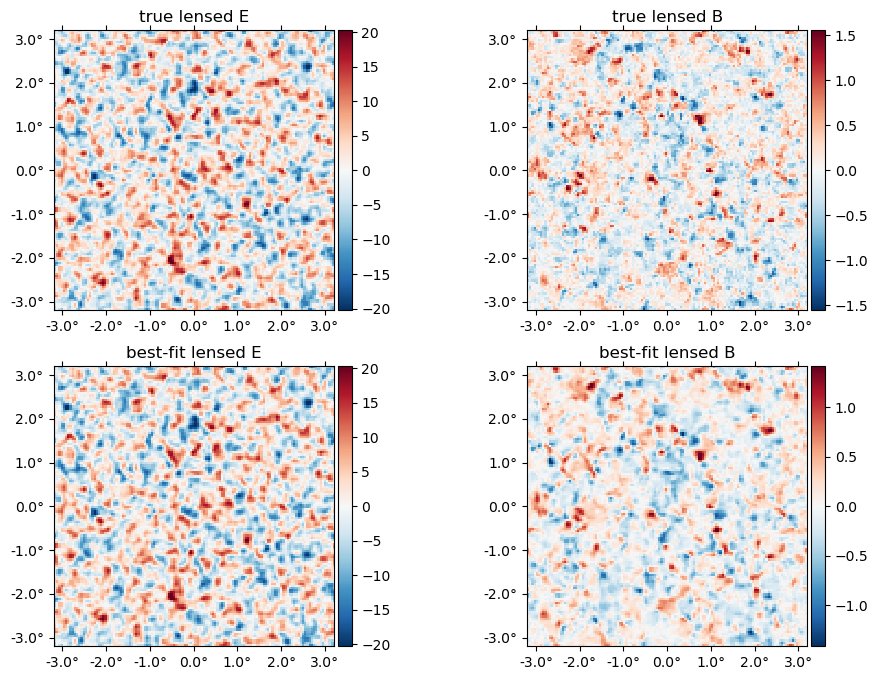# MAP estimation

Here, we give an example of how to compute the joint maximum a posteriori (MAP) estimate of the CMB temperature and polarization fields, $f$, and the lensing potential, $\phi$.

using CMBLensing, PythonPlot

## Compute spectra

First, we compute the fiducial CMB power spectra which generate our simulated data,

Cℓ = camb(r=0.05);

Next, we chose the noise power-spectra:

Cℓn = noiseCℓs(μKarcminT=1, ℓknee=100);

Plot these up for reference,

loglog(Cℓ.total.BB,c="C0")
loglog(Cℓ.unlensed_total.BB,"--",c="C0")
loglog(Cℓ.total.EE,c="C1")
loglog(Cℓ.unlensed_total.EE,"--",c="C1")
loglog(Cℓn.BB,"k:")
legend(["lensed B","unlensed B","lensed E","unlensed E", "noise (beam not deconvolved)"]);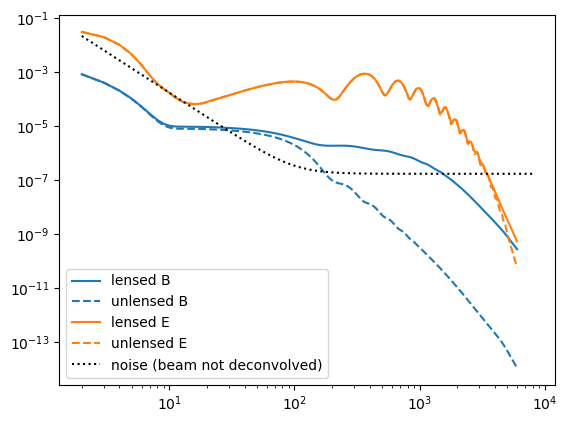## Configure the type of data

These describe the setup of the simulated data we are going to work with (and can be changed in this notebook),

θpix  = 3        # pixel size in arcmin
Nside = 128      # number of pixels per side in the map
pol   = :P       # type of data to use (can be :T, :P, or :TP)
T     = Float32  # data type (Float32 is ~2 as fast as Float64);
Float32

## Generate simulated data

With these defined, the following generates the simulated data and returns the true unlensed and lensed CMB fields, f and f̃ ,and the true lensing potential, ϕ, as well as a number of other quantities stored in the "DataSet" object ds.

(;f, f̃, ϕ, ds) = load_sim(
seed = 3,
Cℓ = Cℓ,
Cℓn = Cℓn,
θpix = θpix,
T = T,
Nside = Nside,
pol = pol,
)

(;Cf, Cϕ) = ds;

## Examine simulated data

The true $\phi$ map,

plot(ϕ, title = raw"true $\phi$");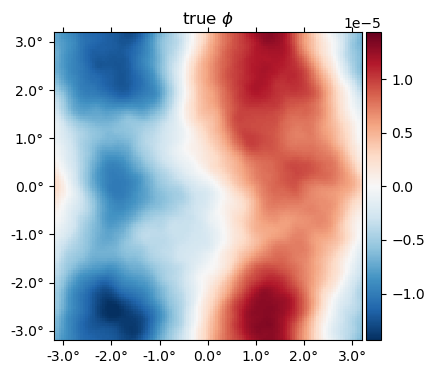The "true" unlensed field, $f$,

plot(f, title = "true unlensed " .* ["E" "B"]);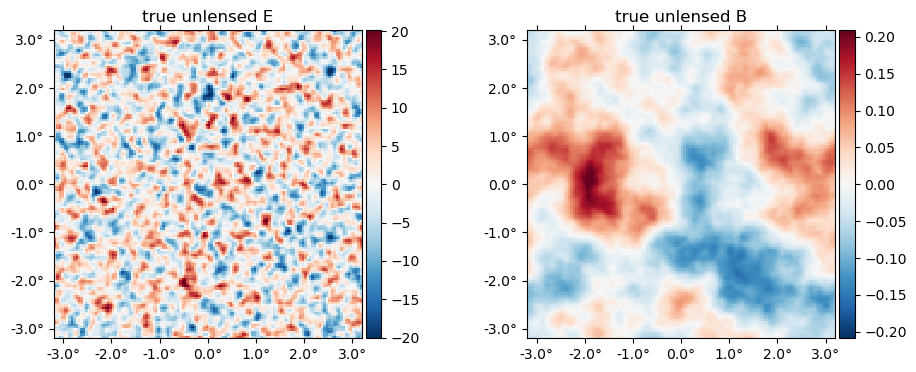And the "true" lensed field,

plot(LenseFlow(ϕ)*f, title = "true lensed " .* ["E" "B"]);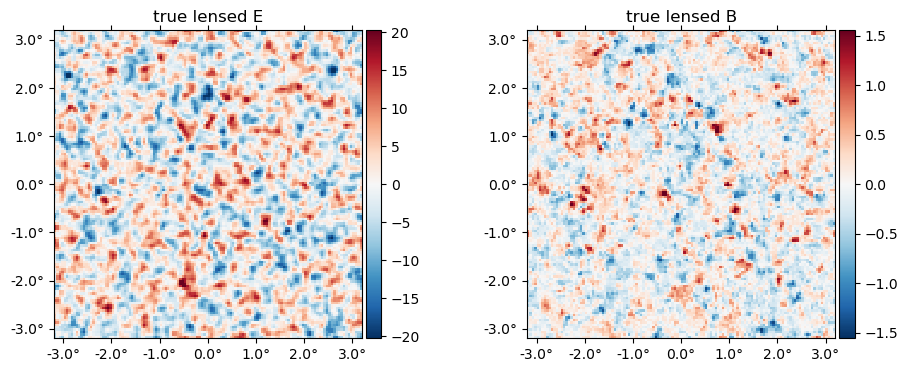The data (stored in the ds object) is basically f̃ with a beam applied plus a sample of the noise,

plot(ds.d, title = "data " .* ["E" "B"]);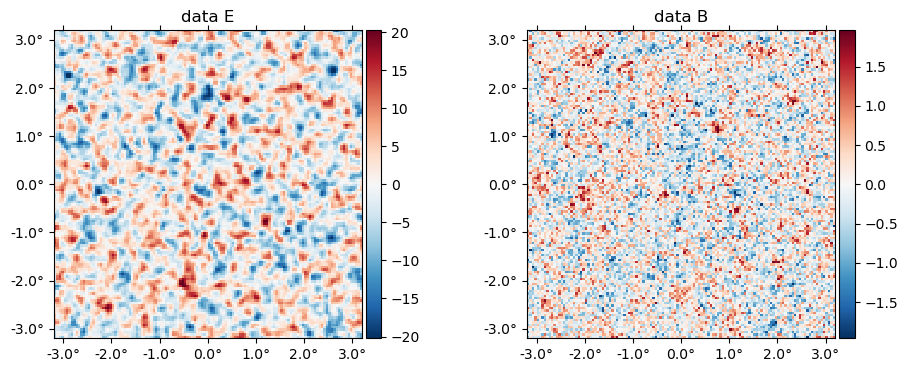# Run the optimizer

Now we compute the maximum of the joint posterior, $\mathcal{P}\big(f, \phi \,\big|\,d\big)$

fJ, ϕJ, history = MAP_joint(ds, nsteps=30, progress=true);
MAP_joint: 100%|████████████████████████████████████████| Time: 0:01:15
step:        30
logpdf:      402508.16
α:           0.4040237
ΔΩ°_norm:    1.6e-06
CG:          2 iterations (0.12 sec)
Linesearch:  16 bisections (0.77 sec)

# Examine results

The history variable gives some info about the run, and more info can be saved by passing history_keys argument to MAP_joint. By default, we get just the value of the posterior, which we can use to check the maximizer has asymptoted to a maximum value:

plot(getindex.(history, :logpdf))
xlabel("step")
ylabel("logpdf");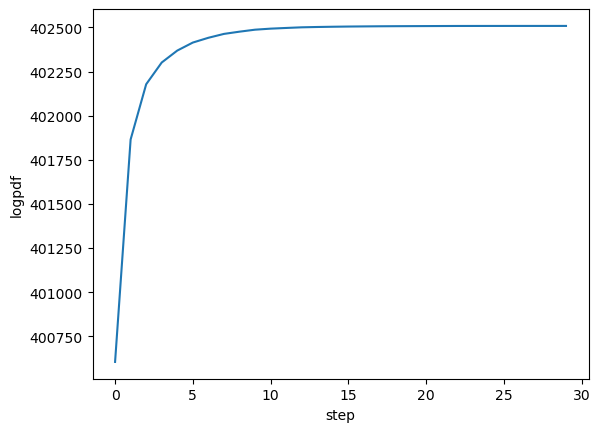Here's the best-fit $\phi$ relative to the truth,

plot(10^6*[ϕ ϕJ], title=["true" "best-fit"] .* raw" $\phi$", vlim=17);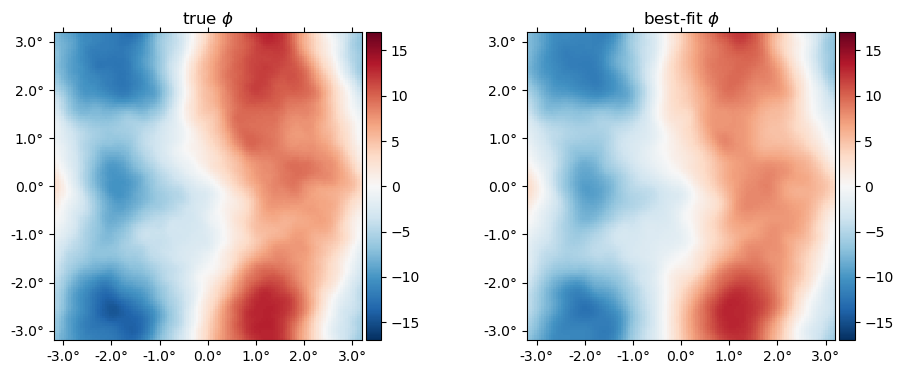Here is the difference in terms of the power spectra. Note the best-fit has high-$\ell$ power suppressed, like a Wiener filter solution (in fact what we're doing here is akin to a non-linear Wiener filter). In the high S/N region ($\ell\lesssim1000$), the difference is approixmately equal to the noise, which you can see is almost two orders of magnitude below the signal.

loglog(ℓ⁴ * Cℓ.total.ϕϕ, "k")
loglog(get_ℓ⁴Cℓ(ϕ))
loglog(get_ℓ⁴Cℓ(ϕJ))
loglog(get_ℓ⁴Cℓ(ϕJ-ϕ))
xlim(80,3000)
ylim(5e-9,2e-6)
legend(["theory",raw"true $\phi$", raw"best-fit $\phi$", "difference"])
xlabel(raw"$\ell$")
ylabel(raw"$\ell^4 C_\ell$");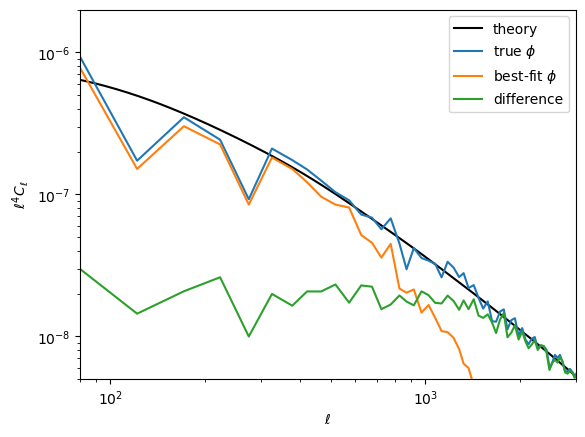The best-fit unlensed fields relative to truth,

plot([f,fJ], title = ["true", "best-fit"] .* " unlensed " .* ["E" "B"]);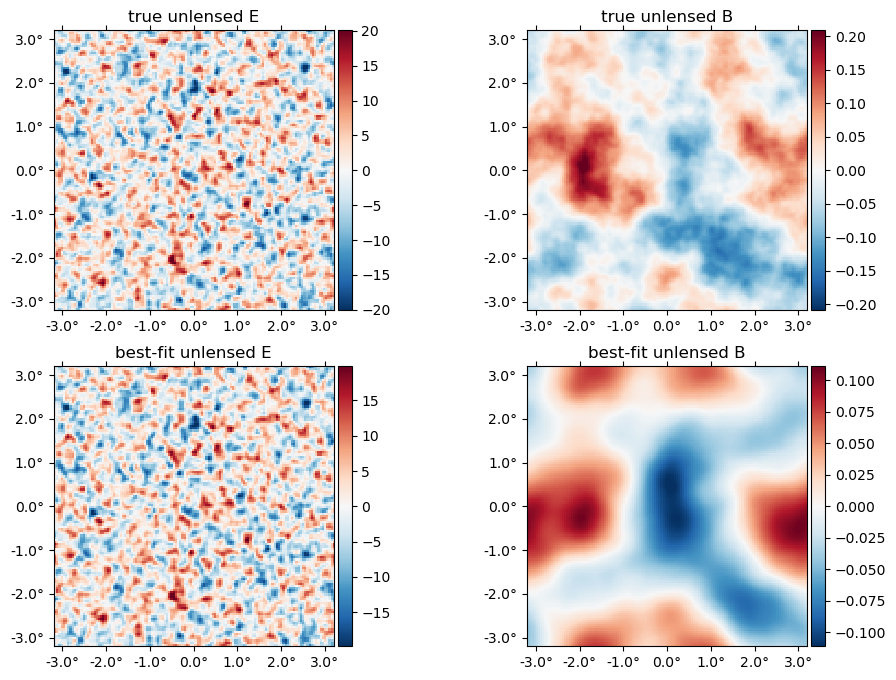The best-fit lensed field (bottom row) relative to truth (top row),

plot([f̃, LenseFlow(ϕJ)*fJ], title = ["true", "best-fit"] .* " lensed " .* ["E" "B"]);